HomeContact
Data Science
Knowledge Distillation
Prashant Brahmbhatt
February 26, 2021
8 min

Remember the time when we had our teachers to help us out in solving difficult problems? Good times. We could have taken our problem to someone who could understand it better and can give a good overview which enabled us then to solve the problem.

I recently found out that same can be done is deep learning as well. I was amazed to know about such a thing. Yes, we can actually teach a model from another model. Superficially it may sound that I am talking about Transfer Learning but I am not. Knowledge Distillation is not to be confused with transfer learning. Both of them are fairly different concepts.

But how is that supposed to happen?

No worries! In this post we are going to discuss the entire process. The explanation is aimed to give the minimal theoretical knowledge but sufficient to understand distillation and implement when needed.

But some for some of you like who like to read the code right away, you can find the code here implemented in Keras. Kudos to Ujjwal Upadhyay for his well written code.

Later we would go over some of the code to see the steps that we discuss in theory first.

The actual paper for this concept can be read here.

But why would we want to learn from another model? If we are to train for learning process can’t we just train on the data itself?

There are couple of situations in which we might want to use distillation, we’ll discuss the advantages later in the post.

## The Teacher and Student

In distillation process, the model which is to learn is termed as the Student model while the model from which we learn is referred to as the Teacher model.

The teacher is usually a more complex model that has more ability and capacity for knowledge from any given data. Large amount of data can be quite easily understood by a model containing hundreds of layers. It is also referred to as ‘Cumbersome’ model. To the gather the same amount of knowledge for a simple model is sometimes impossible due to variations or the large volumes of data.

## The Teaching

We are talking about teaching the model but how is that ‘teaching’ supposed happen technically?

Consider solving a problem from our math book, we take one problem, and try to solve it ourselves. Then we check the back of the book for answers but we find that we got the answer incorrect! What now? The answer helped us to know that what we did was not correct but we’re not really sure what went wrong, we don’t know how close we were, or did we get confused among some steps or what? Now our teacher can help us with that, a simple nudge in the right direction can get us on track. Or if the teacher solves a couple of such problems we can compare our solution with that of our teacher and can get a better idea of the problem.

The same principle is applied in deep learning, the student model is exposed to the answers of the teacher model. We will give the outputs calculated by the cumbersome model to the simpler model.

But isn’t that kinda same as giving it the actual targets, the real ones?

No! And there’s a catch as well. We are not going to provide the final predictions of the teacher model but the logits. Logits here are the outputs obtained before the final activation layer. So the sum of the logit predicition won’t necessarily sum up to 1. If earlier that model was seeing only the targets as `[1,0]` , here it will see something like `[12.7777, -3.99999]` along with the true targets. These scores of the cumbersome model provided in addition are known as soft targets.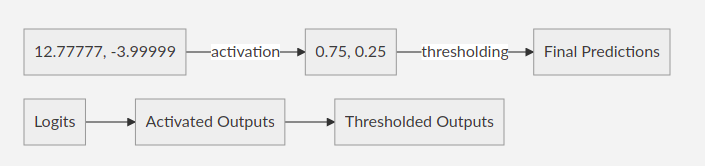When the classes are quite similar or way too different the soft targets will give the model a better idea about such scenarios. Even giving the softmax activated outputs would be slightly better than the targets but logits are what we will work with as proposed in the actual paper.

For the training, we could use the same set that the teacher model used for the training or we could have a separate transfer set if we wish.

During the implementations what we do is just strip out the final activation layer of the teacher model, then get its outputs and just feed them to the student along with the actual targets.

### The Temperature

Temperature is a parameter which is used to tweak the distribution of the soft targets. The general formula for the softmax function is a simpler version of the same formula with temperature: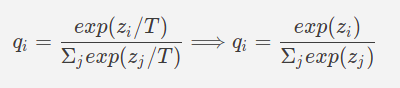The T in the formula stands for that temperature, generally it is taken as 1 while calculating the softmax outputs.

The temperature value effects the sharpness of the distribution of the values. The higher the value of the temperature the softer the distribution becomes. We can observe this behaviour in the below plot for some sample values.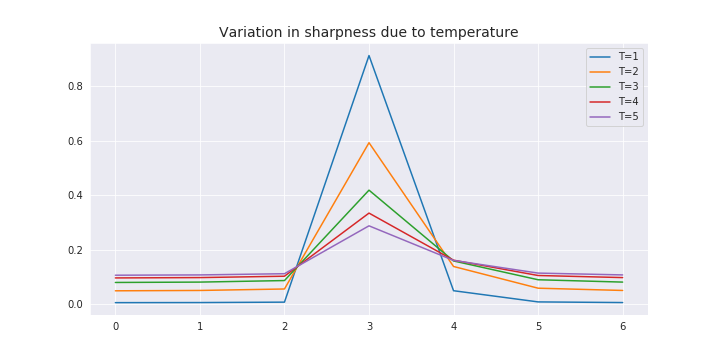As we can see how increasing the temperature has brought down the difference between the sample values.

We can use different values of the temperature for our purposes, just another hyperparameter that we would need to tune.

Although, the different temperature value is only to be used during the training of the student model, once the model is done training the predictions from that model are to be made using the temperature value as 1.

## The Loss Function

We would use the weighted average of two different objective functions.

• The first loss function would be the cross-entropy with the soft targets
• The second loss function would be the cross-entropy with the correct labels.

The loss calculation for the first function has to be computed using the same temperature as was used to compute the logits from the cumbersome model.

### Lambda

The parameter λ is used as the weight parameter for the final loss function. The weighted loss function would be something like the below equation: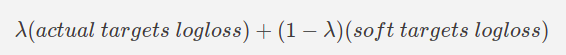Preferably, a higher importance or weight is given to the soft targets which of course, can be controlled to cater to our specific problem. We could use something like 0.1 for value of λ which would mean 90% contribution of soft-target loss while 10% loss from the true target loss. Again, it’s something we would need to play with.

## Ensemble using Distillation

Knowledge Distillation is very suitable for solving problems through ensemble. In some scenarios where the number of classes are large, even a single cumbersome model is not quite able to encompass all the information while also covering the subtle detailed differences.

Using ensemble is one of the workaround, we can create multiple specialised model which only focus on some of the classes which are similar to each other and can be confused with during the predictions.

Once the specialised models are created we can use distillation to encode all of the information from different models into just one model. Rather than using all the models to get ensemble outputs, we could then just use that one distilled model containing the knowledge of all the other models.

## Yays and Nays

#### Pros

There are quite a few advantages of using knowledge distillation, some of them are:

• Highly effective for ensemble techniques.

• Using distillation can save you a lot of space as well, as in the case of ensemble where we could have just one model rather than keeping all the ensemble models to get the outputs.

• Can identify classes not in the data. A simpler model can also give satisfactory results on the classes that it may not have seen at all in the transfer set used in training, provided that the cumbersome model has seen those classes.

• Helpful in getting structure from complex data for simpler models which can have trouble doing that on their own. Sometimes even when a simpler model has enough capacity for large knowledge, it may still struggle with extracting the useful features. The cumbersome model can help it finding those complex features.

• A mystery model about which we don’t have a lot of information can be cloned to an architecture of our choice. This was the use case that we had to face in one of our projects. We had a better model but no idea about its data and other parameters. Our simpler models were struggling. So we used that model to help our simpler model in performing better and it actually worked.

#### Cons

There aren’t really any great downsides of distillations that we noticed but just a couple of caution points.

• It is a little complex to implement correctly. It is not actually a con but something that we just faced. We tried implementing it using Keras. The example problem given in the Keras documentation works but we were not able to make it work using generator to get data from local directories.

• Careful understanding of problem. Before we implement distillation we need to understand our problem statement and how we would fit the distillation and would or would it not help our case.

• May require some more tuning to get balanced performance. Distillation has two more parameters, Lambda and Temperature that we need to tune to get the process working correctly for our use case.

## Getting it Working

Our process for implementation would look something like this,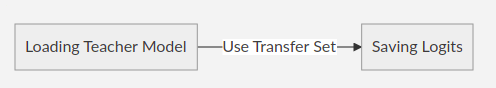We would first create the logits from the teacher model and save them into a numpy file.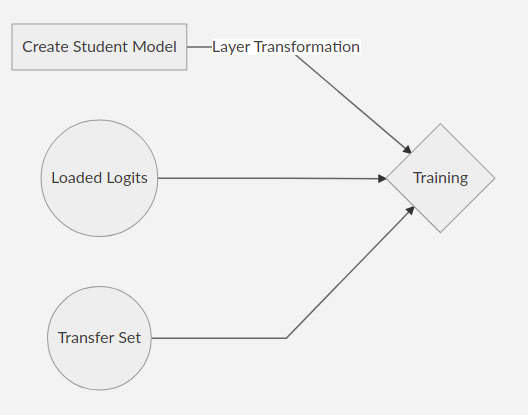Then we will create a student model and modify few last layers and use it for the training with on the transfer set and loading the saved logits.

*The Problem Statement:** Consider we are solving a binary image classification problem, the outputs are supposed to be two. The model that we would consider as the student is an InceptionV3. As the teacher we can assume any large model like VGG16 or ResNet50 or any other for that matter.

Working tensorflow version for this code

The resources and additional utils scripts are available in the repository referenced above.

### Creating True Logits

Getting the requirements

Creating data generators, from the utils (custom) package, for loading the data from local directory. This would return the images, the labels as well as the file names which would help us referencing the logits for respective files.

To get the outputs from the teacher model we would require to strip out the final activation layer. This can be done in two ways.

• For the Sequential API, we can use the `layers.pop()` function which removes the layers from the output side in sequence.
• For the Functional API, we have to create a new model that takes the input from the same source as the teacher but the output is recieved from the second last layer.

You can find the stackoverflow issue for removing layers here.

Now we predict the results from the teacher model and save them to a file. We can optionally save a .csv file as well to see the logits for any sample that we want.

Doing the same for the validation set.

The created logits look like in the image below: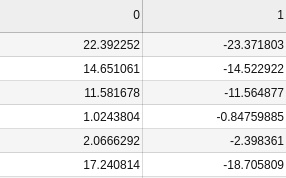## Defining Student

We can define a function to get the student model (InceptionV3).

## The Distillation

Now we will use our saved logits as soft targets to carry out the distillation process.

Getting the imports

Creating the data generators

We can set the temperature for the training

Getting a student model

The tail of the summary would look something like this.

We will now remove the final activation layer from this student model and add a newly created custom layer.

This custom layer in the student model will recieve the previous layer outputs and forms the probabilities as well as the logits, concatenated.

So finally, we will recieve two outputs from the student model, the predicted the targets and predicted soft targets that we would use to compute the soft loss and the target loss.

The new model summary looks like this:

For better understanding we can look at the model plot comparision, before and after this transformation.

Before Transformation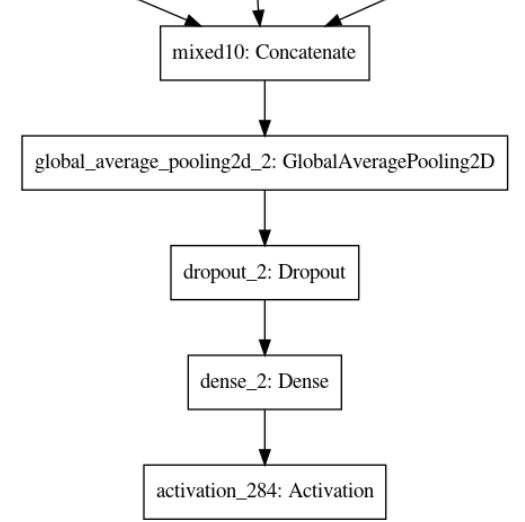After Transformation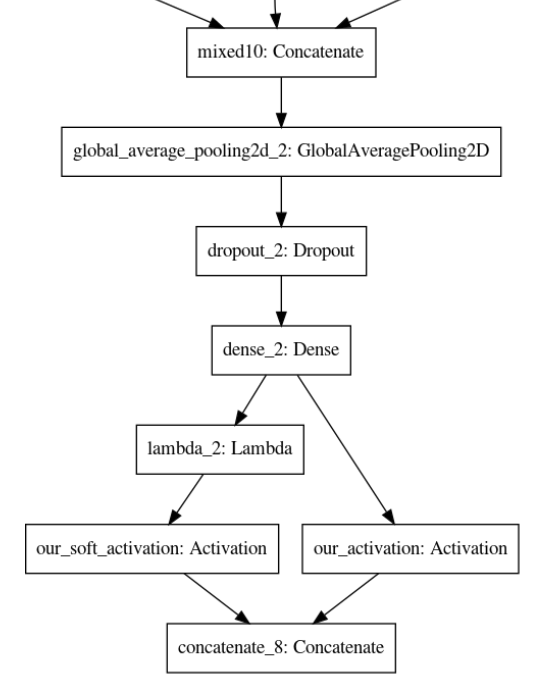Time to create the Knowledge Distillation loss function; from our concatenate output layer, we will recieve 4 outputs (2 times our output classes). The first two would correspond to the true targets and the other two would be for soft targets. We structure the loss function accordingly.

Creating some additional functions for performance check.

Defining the λ for the weightage in loss function computation.

Compiling the model

Fitting the model

Later for using the trained model, if we have to load the weights, first we will have to define the model in the exact same way with all the layer transformations and temperature for it to work.

Alternatively, we can just load the entire model rather than just weights for which we would not have to define anything, making our life easier.

Very Well! here’s all we had to say about Knowledge Distillation, it is truly a brilliant concept and we hope you must have gained something from this post.

We will try to cover more such interesting topics in the near future.

Until next time! Namaste!

## Related Posts

Two Phase Model Training
December 04, 2020
2 min